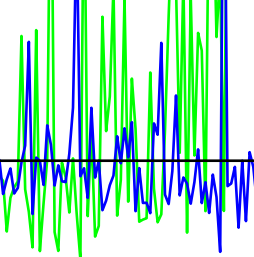# Average nearest neighbor degrees in scale-free networks

Dong Yao, Pim van der Hoorn, Nelly LitvakarXiv
2017
April 19, 2017

#### Abstract

The average nearest  neighbor degree (ANND) of a node of degree k is widely used to measure  dependencies between degrees of neighbor nodes in a network. We formally  analyze ANND in undirected random graphs when the graph size tends to  infinity. The limiting behavior of ANND depends on the variance of the degree  distribution. When the variance is finite, the ANND has a deterministic  limit. When the variance is infinite, the ANND scales with the size of the  graph, and we prove a corresponding central limit theorem in the  configuration model (CM, a network with random connections). As ANND proved  uninformative in the infinite variance scenario, we propose an alternative  measure, the average nearest neighbor rank (ANNR). We prove that ANNR  converges to a deterministic function whenever the degree distribution has  finite mean. We then consider the erased configuration model (ECM), where  self-loops and multiple edges are removed, and investigate the well-known  â€˜structural negative correlationsâ€™, or â€˜finite-size effectsâ€™, that  arise in simple graphs, such as ECM, because large nodes can only have a  limited number of large neighbors. Interestingly, we prove that for any fixed  k, ANNR in ECM converges to the same limit as in CM. However, numerical experiments  show that finite-size effects occur when k scales with n.